# Notes on the Implementation of DenseNet in TensorFlow.

DenseNet(Densely Connected Convolutional Networks) is one of the latest neural networks for visual object recognition. It’s quite similar to ResNet but has some fundamental differences.

With all improvements DenseNets have one of the lowest error rates on CIFAR/SVHN datasets: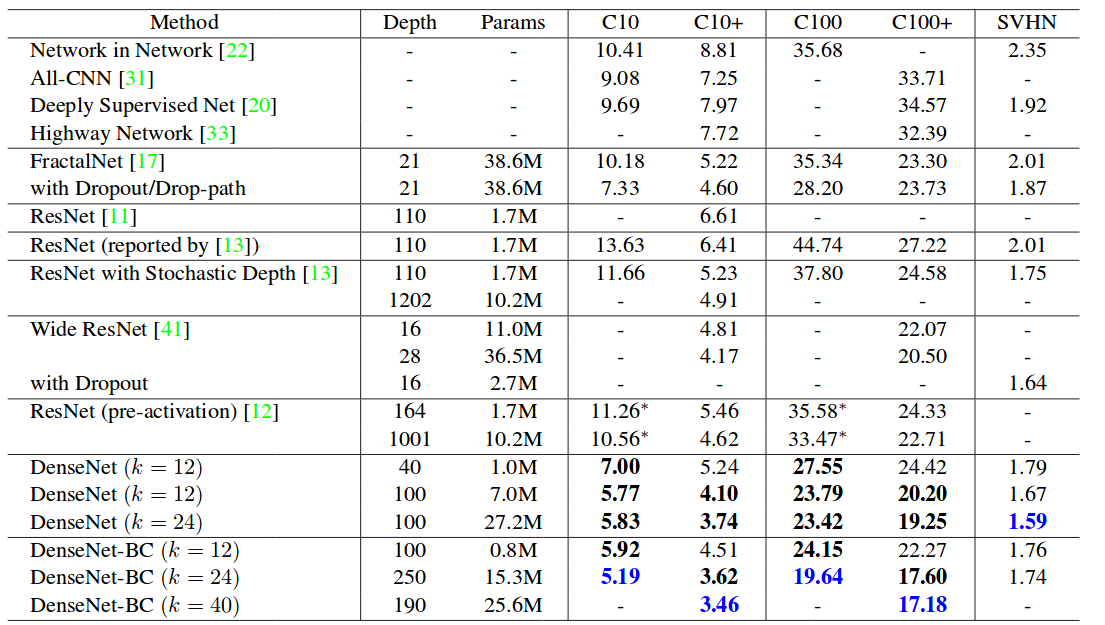Error rates on various datasets(from source paper)

And for ImageNet dataset DenseNets require fewer parameters than ResNet with same accuracy: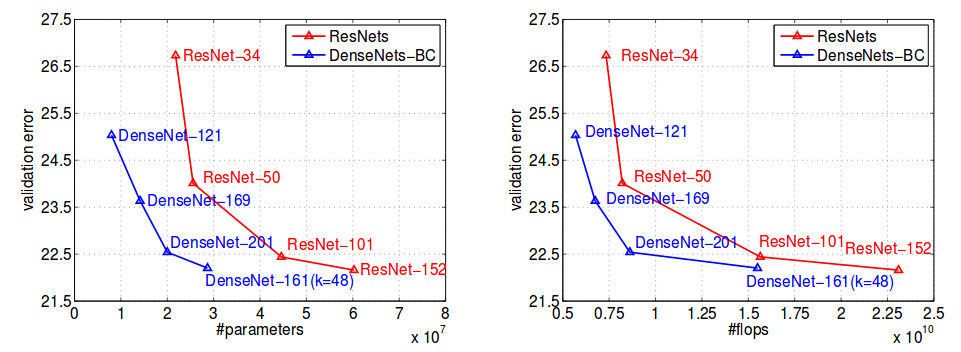Сomparison of the DenseNet and ResNet Top-1 error rates on the ImageNet classification dataset as a function of learned parameters (left) and flops during test-time (right)(from source paper).

This post assumes previous knowledge of neural networks(NN) and convolutions(convs). Here I will not explain how NN or convs work, but mainly focus on two topics:

• Why dense net differs from another convolution networks.
• What difficulties I’ve met during the implementation of DenseNet in tensorflow.

If you know how DenseNets works and interested only in tensorflow implementation feel free to jump to the second chapter or check the source code on GitHub. If you not familiar with any topics but want to get some knowledge — I highly advise you CS231n Stanford classes.

#### Compare DenseNet with other Convolution Networks

Usually, ConvNets work such way:
We have an initial image, say having a shape of (28, 28, 3). After we apply set of convolution/pooling filters on it, squeezing width and height dimensions and increasing features dimension.
So the output from the Lᵢ layer is input to the Lᵢ₊₁ layer. It seems like this: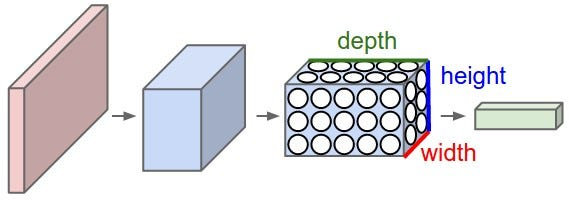source: http://cs231n.github.io/convolutional-networks/

ResNet architecture proposed Residual connection, from previous layers to the current one. Roughly saying, input to the Lᵢ layer was obtained by summation of outputs from previous layers.

In contrast, DenseNet paper proposes concatenating outputs from the previous layers instead of using the summation.
So, let’s imagine we have an image with shape(28, 28, 3). First, we spread image to initial 24 channels and receive the image (28, 28, 24). Every next convolution layer will generate k=12 features, and remain width and height the same.
The output from Lᵢ layer will be (28, 28, 12).
But input to the Lᵢ₊₁ will be (28, 28, 24+12), for Lᵢ₊₂ (28, 28, 24 + 12 + 12) and so on.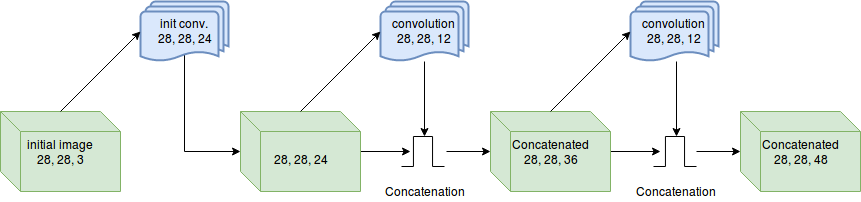Block of convolution layers with results concatenated

After a while, we receive the image with same width and height, but with plenty of features (28, 28, 48).
All these N layers are named Block in the paper. There’s also batch normalization, nonlinearity and dropout inside the block.
To reduce the size, DenseNet uses transition layers. These layers contain convolution with kernel size = 1 followed by 2x2 average pooling with stride = 2. It reduces height and width dimensions but leaves feature dimension the same. As a result, we receive the image with shapes (14, 14, 48).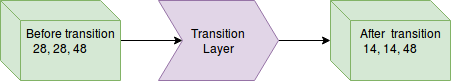Transition layer

Now we can again pass the image through the block with N convolutions.
With this approach, DenseNet improved a flow of information and gradients throughout the network, which makes them easy to train.
Each layer has direct access to the gradients from the loss function and the original input signal, leading to an implicit deep supervision.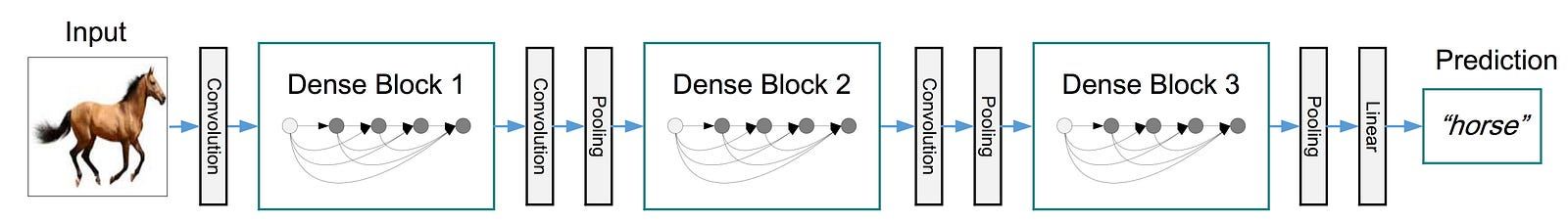Full DenseNet example with 3 blocks from source paper

#### Notes about implementation

In the paper, there are two classes of networks exists: for ImageNet and CIFAR/SVHN datasets. I will discuss details about later one.

First of all, it was not clear how many blocks should be used depends on depth. After I’ve notice that quantity of blocks is a constant value equal to 3 and not depends on the network depth.

Second I’ve tried to understand how many features should network generate at the initial convolution layer(prior all blocks). As per original source code first features quantity should be equal to growth rate(k) * 2 .

Despite that we have three blocks as default, it was interesting for me to build net with another param. So every block was not manually hardcoded but called N times as function. The last iteration was performed without transition layer. Simplified example:

`for block in range(required_blocks):    output = build_block(output)    if block != (required_blocks — 1):        output = transition_layer(output)`

For weights initialization authors proposed use MRSA initialization(as per this paper). In tensorflow this initialization can be easy implemented with variance scaling initializer.

In the latest revision of paper DenseNets with bottle neck layers were introduced. The main difference of this networks that every block now contain two convolution filters. First is 1x1 conv, and second as usual 3x3 conv. So whole block now will be:

`batch norm -> relu -> conv 1x1 -> dropout -> batch norm -> relu -> conv 3x3 -> dropout -> output.`

Despite two conv filters, only last output will be concatenated to the main pool of features.

Also at transition layers, not only width and height will be reduced but features also. So if we have image shape after one block (28, 28, 48) after transition layer, we will get (14, 14, 24).

Where theta — some reduction values, in the range (0, 1).

In case of using DenseNet with bottleneck layers, total depth will be divided by 2. This means that if with depth 20 you previously have 16 3x3 convolution layer(some layers are transition ones), now you will have 8 1x1 convolution layers and 8 3x3 convolutions.

Last, but not least, about data preprocessing. In the paper per channel normalization was used. With this approach, every image channel should be reduced by its mean and divided by its standard deviation. In many implementations was another normalization used — just divide every image pixel by 255, so we have pixels values in the range [0, 1].

At first, I implemented a solution that divides image by 255. All works fine, but a little bit worse, than results reported in the paper. Ok, next I’ve implemented per channel normalization… And networks began works even worse. It was not clear for me why. So I’ve decided mail to the authors. Thanks to Zhuang Liu that answered me and point to another source code that I missed somehow. After precise debugging, it becomes apparent that images should be normalized by mean/std of all images in the dataset(train or test), not by its own only.

And some note about numpy implementation of per channel normalization. By default images provided with data type unit8. Before any manipulations, I highly advise to convert the images to any float representation. Because otherwise, a code may fail without any warnings or errors.

`# without this line next slice assignment will silently fail!# at least in numpy 1.12.0images = images.astype(‘float64’)for i in range(channels):    images[:, :, :, i] = (        (images[:, :, :, i] — self.images_means[i]) /         self.images_stds[i])`

#### Conclusion

DenseNets are powerful neural nets that achieve state of the art performance on many datasets. And it’s easy to implement them. I think the approach with concatenating features is very promising and may boost other fields in the machine learning in the future.

That’s it for now! I hope this post was somehow helpful for you or point to some interesting ideas. Source code can be found in this repository. Thanks for reading!